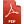# A Study on the Impact of Partition-Induced Dataset Shift on k-fold Cross-Validation - Complementary Material

This Website contains additional material to the SCI2S research paper: A Study on the Impact of Partition-Induced Dataset Shift on k-fold Cross-Validation

J.G. Moreno-Torres, J.A. Sáez, and F. Herrera, A Study on the Impact of Partition-Induced Dataset Shift on k-fold Cross-Validation. IEEE Transactions on Neural Networks 23(8): 1304-1312 (2012)Summary:

## Abstract

J.G. Moreno-Torres, J.A. Sáez, and F. Herrera, A Study on the Impact of Partition-Induced Dataset Shift on k-fold Cross-Validation.

Cross-validation is a very commonly employed technique to evaluate classifier performance. However, it can potentially introduce dataset shift, a harmful factor that is often not taken into account, and which can result in inaccurate performance estimation. This works analyzes both the prevalence and impact of partition-induced covariate shift on different k-fold cross-validation schemes.

From the experimental results obtained we conclude that the degree of partition-induced covariate shift depends on the cross-validation scheme considered. In this way, worse schemes may harm the correctness of a single classifier performance estimation and also increase the needed number of repetitions of cross-validation to reach a stable performance estimation.

## Single-experiment classifier performance analysis results

This section includes the results for the single-experiment classifier performance analysis experiment. There are 4 files, one for each type of partitioning studied (DOB-SCV, DB-SCV, SCV and MS-SCV). Inside each file, you can find the results divided in sheets. Each sheet corresponds to a different partition granularity: 10x1, 5x2 and 2x5. On each sheet, you can then find the test AUC obtained by each method on each dataset.

We also include here the results of the partitions created to obtain "true" classifier estimations. These are presented in a single file, since we use the same data as a reference when studiying all 4 methods. Remember the presented results are classifier performance measured as ROC AUC in the test set.